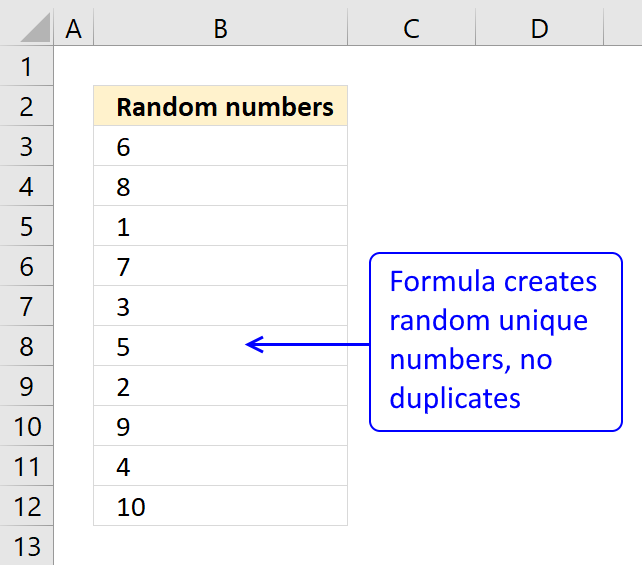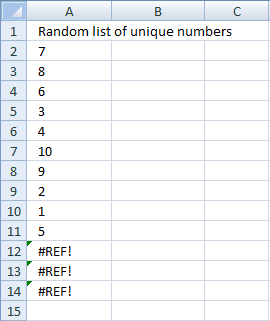Author: Oscar Cronquist Article last updated on February 18, 2018Question: How do I create a random list of unique numbers from say 1 to 10, without using VBA and without enabling iterative calculation in excel options and not using "helper" columns?

The numbers in cell range A2:A11 are random and between 1 and 10.Press function key F9 to generate a new random sequence.

Array formula in A2:

=LARGE(ROW(\$1:\$10)*NOT(COUNTIF(\$A\$1:A1, ROW(\$1:\$10))), RANDBETWEEN(1,11-ROW(A1)))

The following article demonstrates how to sort values in a column in a random order, using an array formula:

Sort a list in random order in excel

Overview The array formula in cell range C2:C6 creates a random list from the values in cell range A2:A6. Array […]

How to enter an array formula

1. Select cell A2
2. Copy / Paste above array formula to formula bar3. Press and hold CTRL + SHIFT
4. Press Enter
5. Release all keys

How to enter an array formula

Array formulas allows you to do advanced calculations not possible with regular formulas.

Copy cell A2 and paste down as far as needed.

### Explaining array formula in cell A2

Step 1 - Create an array

ROW(\$1:\$10) creates this array {1, 2, 3, 4, 5, 6, 7, 8, 9, 10}

Step 2 - Create a criterion to avoid duplicate numbers

COUNTIF(\$A\$1:A1, ROW(\$1:\$10)) makes sure no duplicate numbers are created. The formula has both absolute and relative cell references (\$A\$1:A1). When the formula are copied down to cell A3 the cell reference changes to \$A\$1:A2. The value in cell A2 can´t be randomly selected again.

In cell A2, COUNTIF(\$A\$1:A1, ROW(\$1:\$10)) creates this array: {0, 0, 0, 0, 0, 0, 0, 0, 0, 0)

How to use the COUNTIF function

Counts the number of cells that meet a specific condition.

Step 3 - Create a new dynamic array

ROW(\$1:\$10)*NOT(COUNTIF(\$A\$1:A1, ROW(\$1:\$10))) creates this array in cell A2: {1, 2, 3, 4, 5, 6, 7, 8, 9, 10}

If the array formula  randomly selects the number 2 in cell A2, the formula in cell A3 creates this array: {1, 0, 3, 4, 5, 6, 7, 8, 9, 10}

Number 2 can´t be selected anymore.

Step 4 - Calculate the number range in Randbetween(bottom, top)

The bottom value is always 1. The top value changes depending on current cell.

In cell A2 the top value is 10.

In cell A3 the top value is 9

and so on..

Formula in cell A2: 11-ROW(A1) equals 10. (11-1=10)

Formula in cell A3: 11-ROW(A2) equals 9. (11-2=9)

and so on..

Step 5 - Create a random number

=LARGE(ROW(\$1:\$10)*NOT(COUNTIF(\$A\$1:A1, ROW(\$1:\$10))), RANDBETWEEN(1,11-ROW(A1)))

RANDBETWEEN(1,11-ROW(A1))

becomes

RANDBETWEEN(1,11-1)

becomes

RANDBETWEEN(1,10)

and returns a random number between 1 and 10.

How to generate random numbers and text

What's on this page Random numbers with a decimal Random negative numbers with two decimals Random negative numbers with three […]

Step 6 - Select a random number in array

=LARGE(ROW(\$1:\$10)*NOT(COUNTIF(\$A\$1:A1, ROW(\$1:\$10))), RANDBETWEEN(1,11-ROW(A1)))

becomes

=LARGE({1, 2, 3, 4, 5, 6, 7, 8, 9, 10}, RANDBETWEEN(1,10))

becomes

=LARGE({1, 2, 3, 4, 5, 6, 7, 8, 9, 10}, random_number) and returns a random number between 1 and 10.

How to use the SMALL function

The SMALL function lets you extract a number in a cell range based on how small it is compared to the other numbers in the group.

### How to customize array formula to your excel work sheet

If your list starts at F3 change \$A\$1:A1 to \$F\$2:F2 in the above array formula. To change the numbers from 1 to 10 to, for example, 2 to 12, change \$1:\$10 to \$2:\$12 also in the above array formula.
Press F9 to generate a new random list of unique numbers.